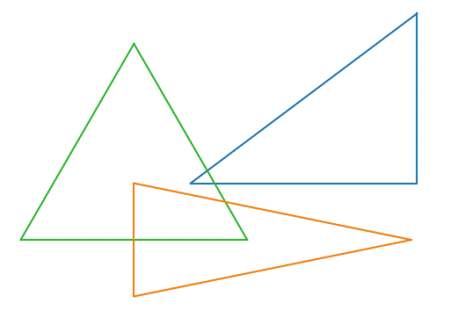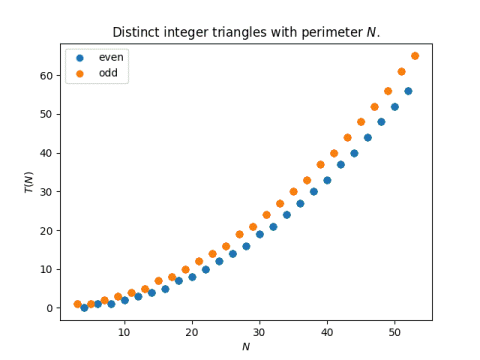# Counting triangles with integer sides

Let T(N) be the number of distinct (non-congruent) triangles with integer sides and perimeter N.  For example, T(12) = 3 because there are three distinct triangles with integer sides and perimeter 12. There’s the equilateral triangle with sides 4 : 4 : 4, and the Pythagorean triangle 3 : 4 : 5. With a little more work we can find 2 : 5 : 5.The authors in  developed an algorithm for finding T(N). The following Python code is a direct implementation of that algorithm.

```    def T(N :int):
if N < 3:
return 0
base_cases = {4:0, 6:1, 8:1, 10:2, 12:3, 14:4}
if N in base_cases:
return base_cases[N]
if N % 2 == 0:
R = N % 12
if R < 4:
R += 12
return (N**2 - R**2)//48 + T(R)
if N % 2 == 1:
return T(N+3)
```

If you’re running a version of Python that doesn’t support type hinting, just delete the `:int` in the function signature.

Since this is a recursive algorithm, we should convince ourselves that it terminates. In the branch for even `N`, the number R is an even number between 4 and 14 inclusive, and so it’s in the `base_cases` dictionary.

In the odd branch, we recurse on `N+3`, which is a little unusual since typically recursive functions decrease their argument. But since `N` is odd, `N+3` is even, and we’ve already shown that the even branch terminates.

The code `(N**2 - R**2)//48` raises a couple questions. Is the numerator divisible by 48? And if so, why specify integer division (`//`) rather than simply division (`/`)?

First, the numerator is indeed divisible by 48. N is congruent to R mod 12 by construction, and so NM is divisible by 12. Furthermore,

N² – R² = (NR)(N + R).

The first term on the right is divisible by 12, so if the second term is divisible by 4, the product is divisible by 48.  Since N and R are congruent mod 12, N + R is congruent to 2R mod 12, and since R is even, 2R is a multiple of 4 mod 12. That makes it a multiple of 4 since 12 is a multiple of 4.

So if (N² – R²)/48 is an integer, why did I write Python code that implies that I’m taking the integer part of the result? Because otherwise the code would sometimes return a floating point value. For example, `T(13)` would return 5.0 rather than 5.

Here’s a plot of T(N).J. H. Jordan, Ray Walch and R. J. Wisner. Triangles with Integer Sides. The American Mathematical Monthly, Vol. 86, No. 8 (Oct., 1979), pp. 686-689

## One thought on “Counting triangles with integer sides”

1. Jack Kennedy

No need to recurse!

def T(n):
m = n if n % 2 == 0 else n+3
return round(m*m / 48)